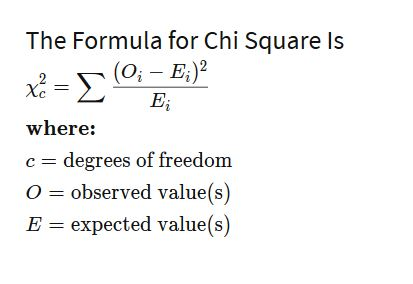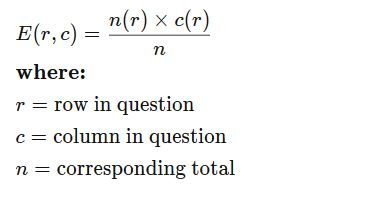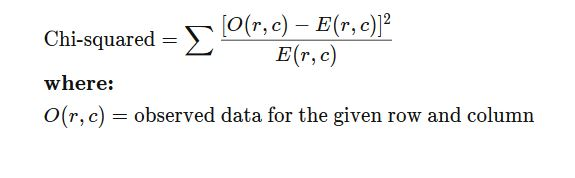### Chi Square Test

• date 20th July, 2019 |
• by Prwatech |

# Chi Square Test Tutorial

Chi Square test tutorial, Welcome to the world of chi Square test in Data science. Now, we are going to cover the introduction of chi square test tutorial. Along with this, we will study various uses of it and formula to calculate with example.

Are you the one who is looking for the best platform to learn Data science tutorials? Or Are you the one who is dreaming to become an expert data scientist? Then stop dreaming yourself, start taking Data Science training from Prwatech, who can help you to guide and offer excellent training with highly skilled expert trainers with the 100% placement. Follow the below mentioned chi square test in data science and enhance your skills to become pro Data Scientist.

## What is a Chi Square Test?

A chi square (χ2) test is a test that measures how expectations are compared to actual observed data. The data used in calculating a chi square test must be random, raw, mutually exclusive, drawn from independent variables, and drawn from a large enough sample. It is often used in hypothesis testing.

Example: The results of tossing a coin 1000 times meet these criteria

### Why we need a Chi Square Test?

There are two main types of chi-square tests:

The test of independence, that asks a question of relationship, like, “Is there a relationship between gender and SAT scores?”

The goodness-of-fit test, that asks something like “If a coin is tossed 1000 times, will it head 500 times and tails 500 times?”

For these kinds of tests, degrees of freedom are used to identify if a specific null hypothesis can be rejected based on the total number of variables and samples taken in the experiment. For example, Consider, employees and their vehicle was chosen to travel home, a sample size of 30 or 40 employees is likely not large enough to create significant amount data. Getting the same or similar results from a study using a sample size of 400 or 500 employees is more valid.

## Chi Square Test Formula## Chi Square Test Example

Imagine a random poll was taken across 20,000 different voters, both male and female. The people who responded were classified according to their gender and whether they were republican, democrat or independent.

Imagine a grid with the columns labeled republican, democrat, and independent, and two rows labeled as male and female. Assume the data from the 20,000 respondents is as follows:

 Republican Democrat Independent Total Male 4000 3000 1000 8000 Female 5000 6000 1000 12000 Total 9000 9000 2000 20000

Step 1) Find the expected frequencies.

These are calculated for each “cell” in the grid. As such there are two categories of gender and three categories of political view, there are total six expected frequencies. The formula for the expected frequency is:Hence:

• E(1,1) = (9000*8000)/20000 =3600
• E(1,2) =(9000*8000)/20000 =3600
• E(1,3) =(2000*8000)/20000 =800
• E(2,1) =(9000*12000)/20000 =5400
• E(2,2) =(9000*12000)/20000 =5400
• E(2,3) =(2000*12000)/20000 =1200

Step 2) These values are the used to calculate the chi squared statistic using the following formula:• O(1,1)=(4000-3600)²/3600 = 44.44
• O(1,2)=(3000-3600)²/3600 = 100
• O(1,3)=(1000-800)²/800 = 50
• O(2,1)=(5000-5400)²/5400 = 29.63
• O(2,2)=(6000-5400)²/5400 = 66.66
• O(2,3)=(1000-1200)²/1200 = 33.33

Chi-squared = 324.66

The chi squared statistic then equals to the sum of these value, or 324.66. We can then look at a chi squared statistic table to see, given the degrees of freedom in our set-up, whether the result is statistically significant or not.

We hope you have understood the basics of the Chi square test tutorial and its formula with examples in data science. Planning towards becoming a skilled expert in Data Science? If so, be a part of the Prwatech learning program of Data Science Training in Bangalore.

### Quick Support